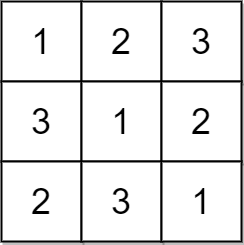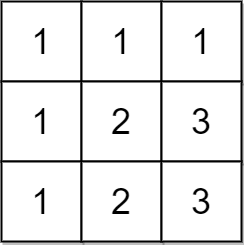2133. Check if Every Row and Column Contains All Numbers

#### QUESTION:

An n x n matrix is valid if every row and every column contains all the integers from 1 to n (inclusive).

Given an n x n integer matrix matrix, return true if the matrix is valid. Otherwise, return false.

Example 1:``````Input: matrix = [[1,2,3],[3,1,2],[2,3,1]]
Output: true
Explanation: In this case, n = 3, and every row and column contains the numbers 1, 2, and 3.
Hence, we return true.
``````

Example 2:``````Input: matrix = [[1,1,1],[1,2,3],[1,2,3]]
Output: false
Explanation: In this case, n = 3, but the first row and the first column do not contain the numbers 2 or 3.
Hence, we return false.
``````

Constraints:

``````n == matrix.length == matrix[i].length
1 <= n <= 100
1 <= matrix[i][j] <= n
``````

#### SOLUTION:

``````class Solution {
func checkValid(_ matrix: [[Int]]) -> Bool {
for ma in matrix {
if Set(ma).count != ma.count{
return false
}
}
for indexI in 0..<matrix.count {
var tmpArr: [Int] = []
for indexJ in 0..<matrix.count {
tmpArr.append(matrix[indexJ][indexI])
}
if Set(tmpArr).count != matrix[indexI].count{
return false
}
}
return true

}
}
``````